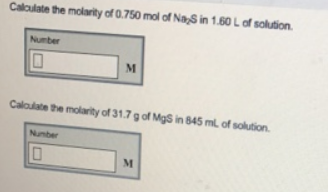# Problem: Calculate the molarity of 0.750 mol of Na2S in 1.60 L of solution. Caloulate the molarity of 31.7 g of MgS in 845 mL of solution.

###### FREE Expert Solution
94% (114 ratings)###### Problem Details

Calculate the molarity of 0.750 mol of Na2S in 1.60 L of solution.

Caloulate the molarity of 31.7 g of MgS in 845 mL of solution.